Function Repository Resource:

# MagicSquare

Get a magic square for any dimension

Contributed by: Onkar Singh
 ResourceFunction["MagicSquare"][n] gives a magic square of dimension n.

## Details

A magic square is an n×n square array of the numbers 1,2,,n2, arranged so that the sum of the numbers in a row, column or main diagonal is constant.

## Examples

### Basic Examples (1)

Get a 3×3 magic square (also known as the Lo Shu square):

 In:=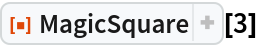Out=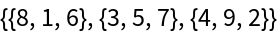### Scope (2)

Generate a 6×6 magic square:

 In:=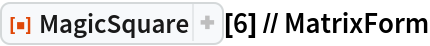Out=An 8×8 magic square:

 In:=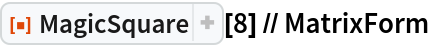Out=### Properties and Relations (2)

Visualize the sums within a 5×5 magic square:

 In:=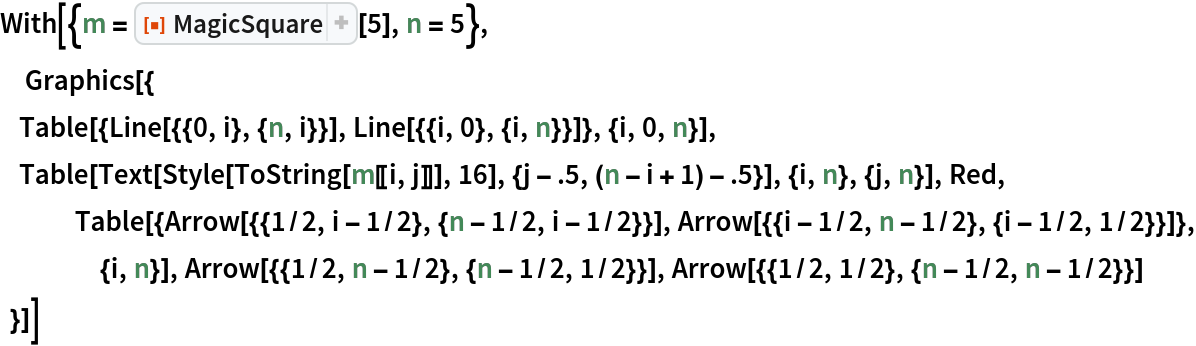Out=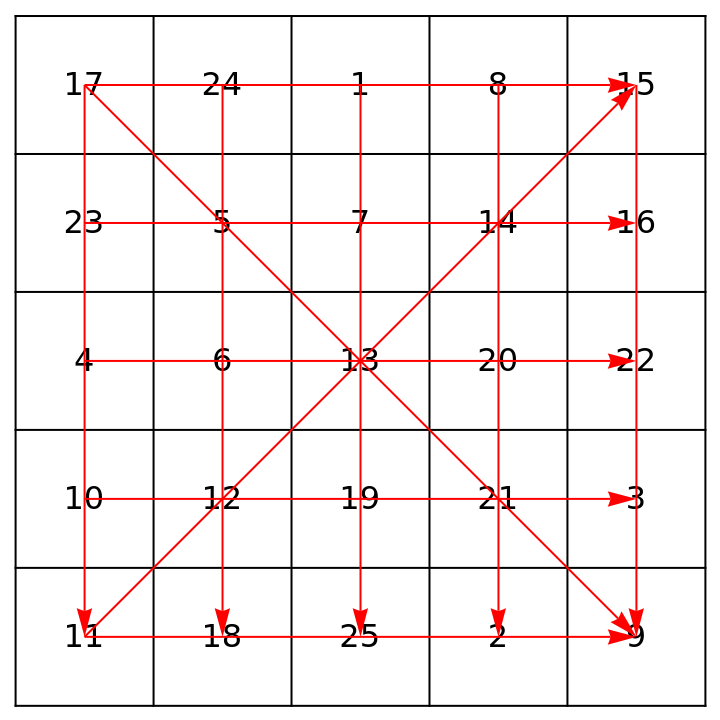Check that the row, column, main diagonal and antidiagonal sums all agree, and are equal to the magic constant:

 In:=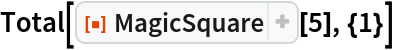Out=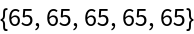In:=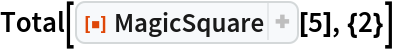Out=In:=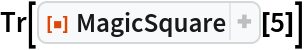Out=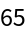In:=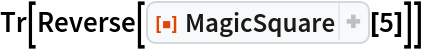Out=In:=Out=## Requirements

Wolfram Language 11.3 (March 2018) or above

## Version History

• 1.1.0 – 24 August 2021
• 1.0.0 – 20 February 2019